Courses

# Doc: Effect of Temperature Change Class 11 Notes | EduRev

## Class 11 : Doc: Effect of Temperature Change Class 11 Notes | EduRev

The document Doc: Effect of Temperature Change Class 11 Notes | EduRev is a part of the Class 11 Course Chemistry Class 11.
All you need of Class 11 at this link: Class 11

Effect of Temperature on Equilibrium constant

VAN'T HOFF EQUATION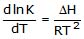where K is the equilibrium constant, T is temperature, ΔH is the enthalpy of the reaction and R is the gas constant. It provides means to detemine how the equilibrium constant for a reaction or process will vary with temperature.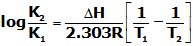Ex. H2O(l)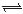H (aq)+ + OH-(aq) , ΔH= 13.7 kcal/mol , Keq at 25°C =10-14. Calculate Keq at 60°C ?

Sol.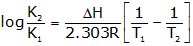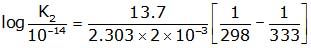K2= 1.26×10-12

LeChâtelier principle

If a system at equilibrium is subjected to a change of pressure, temperature, or the number of moles of a substance, there will be a tendency for a net reaction in the direction that tends to reduce the effect of this change.

1. EFFECT OF CHANGE IN CONCENTRATION

The change in concentration can affect gaseous systems or liquid solution systems only. However this does not affect the solid and pure liquid systems since their active masses are always taken as unity. By using le Chatelier's principle, the effect of change in concentration on systems at equilibrium can be explained as follows:

1. When the concentration of reactant(s) is increased, the system tries to reduce their concen tration by favoring the forward reaction.

2. When the concentration of product(s) is increased, the system tries to reduce their concentration by favoring the backward reaction.

3. When the concentration of reactant(s) is decreased, the system tries to increase their concentration by favoring the backward reaction.

4. When the concentration of product(s) is decreased, the system tries to increase their concentration by favoring the forward reaction.

When the concentration of reactants is increased, the number of effective collisions between them increases which in turn increases the rate of forward reaction. Thus the forward reaction is more favored over the backward reaction until the new equilibrium is established. At this new equilibrium, the rates of both forward and backward reactions become equal again and the reaction quotient becomes approximately equal to the equilibrium constant.

Remember that small changes in concentration do not affect the equilibrium constant.

For decomposition reaction , PCl5(g)PCl3(g) + Cl2(g)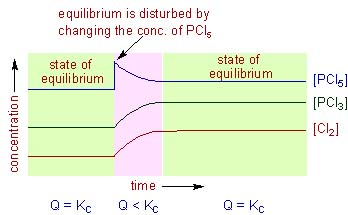Let the concentration of PCl5 is doubled to disturb the equilibrium. This will change the reaction quotient, Q to:

After disturbing the equilibrium, the value of Q becomes less than KC. In order to restore the Q value to KC, the concentration of PCl5 must be decreased while the concentrations of PCl3 and Cl2 are to be increased. This is achieved by favoring the forward reaction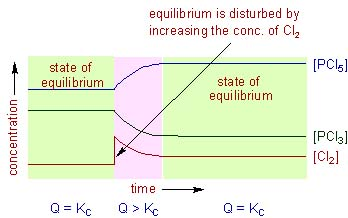The forward reaction is also favored by removing the products from the reaction mixture (decrease in the concentration of products). Upon removal of products, the rate of forward reaction becomes greater than that of backward reaction momentarily. This will also decrease the reaction quotient. Hence the system tries to reestablish the equilibrium by converting more reactants to products so as to make the rates of both forward and backward reactions become equal again.

For example, in case of the decomposition of PCl5, if the concentration of Cl2 is increased by two times at equilibrium, the Q value becomes greater than the KC value.

Hence the system tries to restore the value of Q to KC again. The backward reaction is favored to decrease the concentration of Cl2. However the concentration of PCl5 also decreases automatically while the concentration of PCl5 increases while doing so.

2. EFFECT OF CHANGE IN PRESSURE

The change in pressure only affects the equilibrium of systems involving at least one gas. The le Chatelier's principle can be applied to understand the effect of change in pressure on the systems at equilibrium as follows.

1. When the partial pressure of any of the gaseous reactants or of the products is increased, the position of equilibrium is shifted so as to decrease its partial pressure. This is usually achieved by favoring the reaction in which there is decrease in the number of moles of gaseous components.

2. When the partial pressure of any of the gaseous reactants or of the products is decreased, the position of equilibrium is shifted so as to increase its partial pressure. This can be achieved by favoring that reaction in which there is increase in the number of moles of gaseous components.

However, it is not always correct to say that the equilibrium is shifted whenever there is a change in the total pressure of the system. The equilibrium is not always disturbed upon changing the pressure of the entire system. It is only disturbed whenever there is a change in the partial pressure of any or all of the gaseous reactants or products in the equilibrium for which the Dng ¹ 0. Where Dng = (no. of moles of gaseous products) - (no.of moles of gaseous reactants) Strictly speaking, the equilibrium is only shifted when the ratio of product of partial pressures of products to the product of partial pressures of reactants i.e., the reaction quotient in terms of partial pressures, Qp is disturbed. The position of equilibrium is shifted so as to make Qp become equal to the value of Kp again.

The Qp can be changed in the following cases:

1. By adding or removing any gaseous reactant or product at constant volume. The effect is same as changing the concentration as explained above.

2. By changing the volume of the system (or in other words by changing the pressure of entire system) at equilibrium for which the Dng ¹ 0. In this case, however, the pressure of the entire system is also changed.

For the decomposition of PCl5, the Kp can be written as: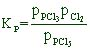For this reaction Dng = (1 + 1)-(1) = 1

Hence for this reaction, if the pressure of the system is increased by 2 times by halving the volume, the reaction quotient, Qp is doubled. In order to restore back the Qp value again to Kp, the denominator value i.e., the partial pressure of PCl5must be increased. This is only achieved by favoring the forward reaction in which less number of gaseous products are formed. i.e., Two moles of products (PCl3 and Cl2) are converted to one mole of reactant (PCl5)

Therefore we can say, if the pressure of the system is increased, the system tries to decrease it by favoring the reaction in the direction of decreasing the number of moles of gaseous components.

(A) At constant volume :there is no effect of adding inert gas on the state of equilibrium at constant volume.

(B) At constant pressure : On Adding of inert gas At constant pressure, the reaction proceeds in that direction where the sum of stoichiometric coefficient of gaseous components is greater.

4. EFFECT OF CHANGE IN TEMPERATURE

The effect of temperature can be understood by using le Chatelier's principle as follows:

1. Increase in the temperature of the system favors the endothermic reaction. The increase in temperature increases the amount of heat in the system. Hence it tries to remove the excess of heat by favoring that reaction in which heat is absorbed i.e., the endothermic reation.

2. Decrease in the temperature of the system favors the exothermic reaction. In this case, the temperature is decreased by removing the heat content from the system. Hence the system tries to restore the temperature back by favoring the exothermic reaction i.e., the reaction in which the heat is liberated.

It is very important to note that, during the change in temperature, the system establishes a new equilibrium for which the value of equilibrium constant is different from the original constant i.e., the equilibrium constant depends on the temperature.

EFFECT OF CATALYST

A catalyst has no effect on the position of the equilibrium since it increases not only the rate of forward reaction but also the rate of backward reaction. However it does help the system to reach the equilibrium faster.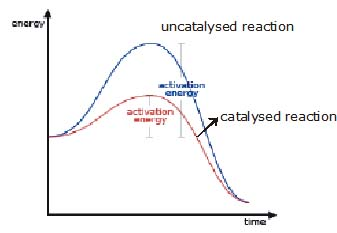Offer running on EduRev: Apply code STAYHOME200 to get INR 200 off on our premium plan EduRev Infinity!

## Chemistry Class 11

136 videos|245 docs|180 tests

,

,

,

,

,

,

,

,

,

,

,

,

,

,

,

,

,

,

,

,

,

;